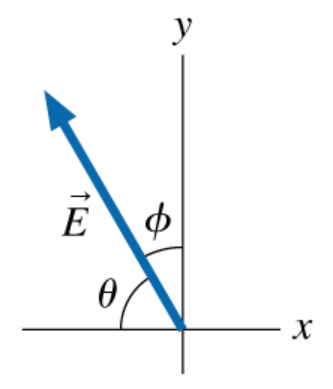# Problem: Part A: What is the x-component of vector E of the figure in terms of the angle θ and the magnitude E ? (Express your answer in terms of the variables θ and E )Part B: What is the y-component of vector E⃗ of the figure in terms of the angle θ and the magnitude E ? (Express your answer in terms of the variables θ and E )Part C: For the same vector, what is the x-component in terms of the angle ϕ and the magnitude E ? (Express your answer in terms of the variables ϕ and E )Part D: For the same vector, what is the y-component in terms of the angle ϕ and the magnitude E ? (Express your answer in terms of the variables ϕ and E )

###### FREE Expert Solution

Vector Components:

Part A

Ex = E(-cosθ), since cosθ is always negative when θ is above the negative x-axis.

Ex = -Ecosθ

89% (73 ratings)###### Problem DetailsPart A: What is the x-component of vector of the figure in terms of the angle θ and the magnitude E ? (Express your answer in terms of the variables θ and E )

Part B: What is the y-component of vector E⃗ of the figure in terms of the angle θ and the magnitude E ? (Express your answer in terms of the variables θ and E )

Part C: For the same vector, what is the x-component in terms of the angle ϕ and the magnitude E ? (Express your answer in terms of the variables ϕ and E )

Part D: For the same vector, what is the y-component in terms of the angle ϕ and the magnitude E ? (Express your answer in terms of the variables ϕ and E )